Homework Help Question & Answers

# There are two multiplexers in the following circuit. The three ports A, B, C are inputs,...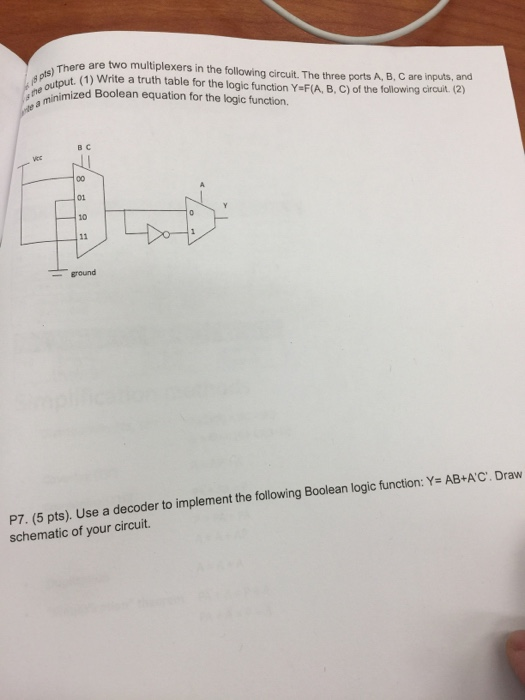There are two multiplexers in the following circuit. The three ports A, B, C are inputs, and s) uput(1) Write a truth table for the logic function Y F(A, B, C) of the following cirouit (2) ne inimized Boolean equation for the logic function. B C 01 10 ground P. (5 pts). Use a decoder to implement the following Boolean logic function: Y= AB+AC. Draw schematic of your circuit.

#### Homework Answers

Answer #1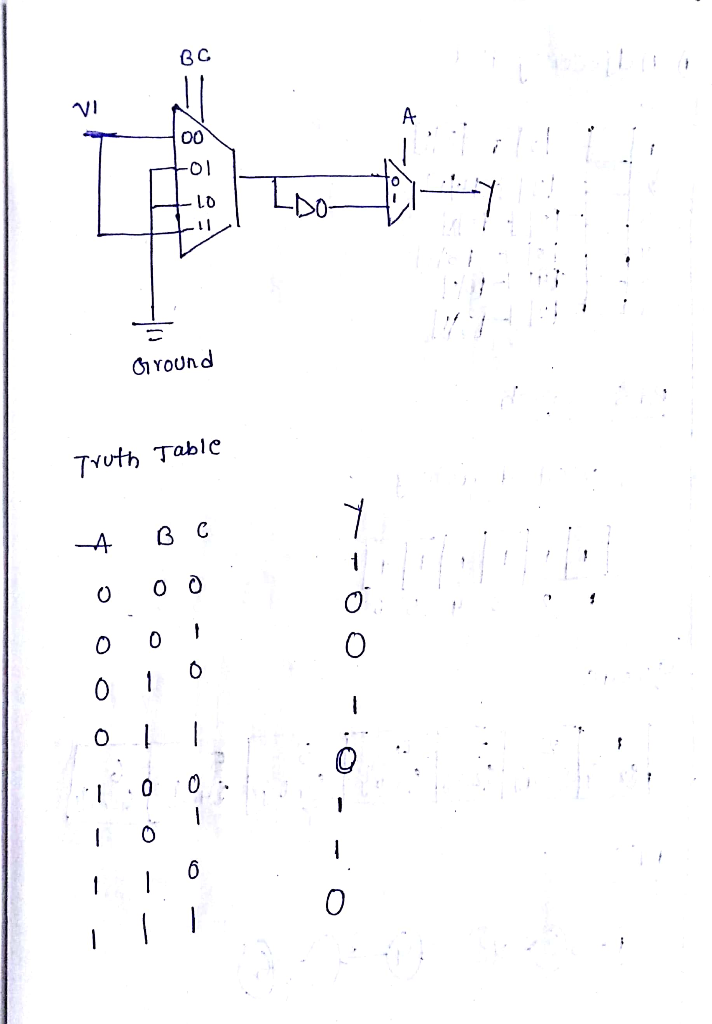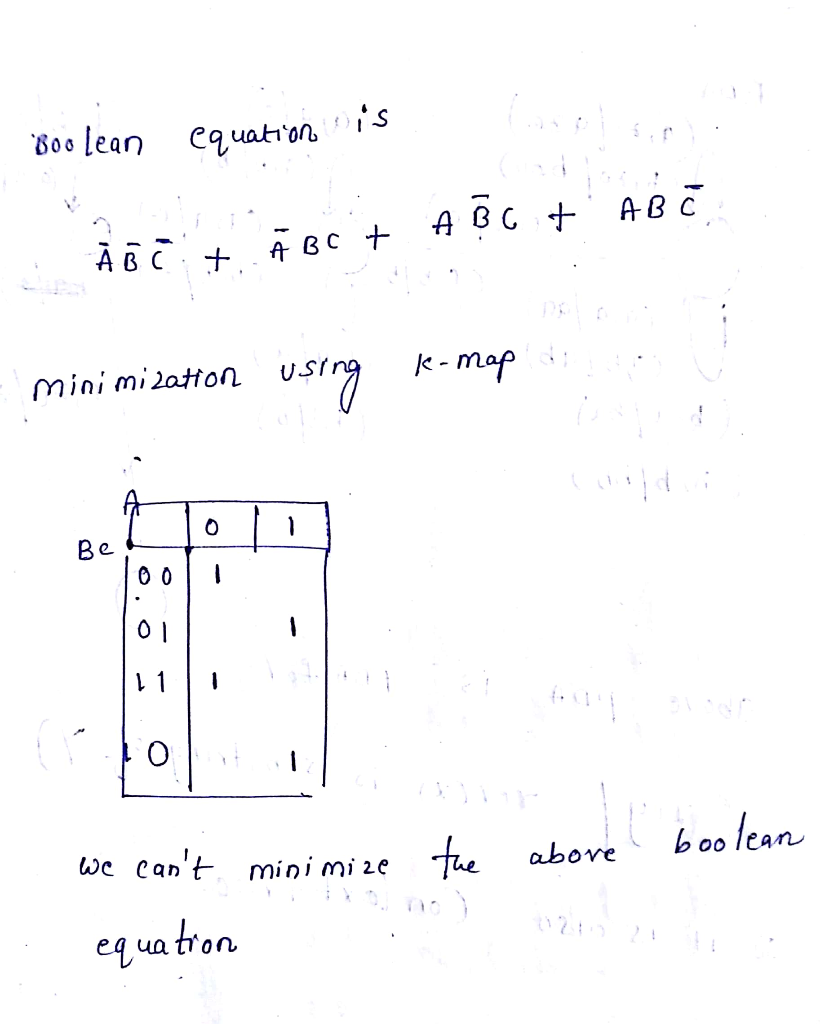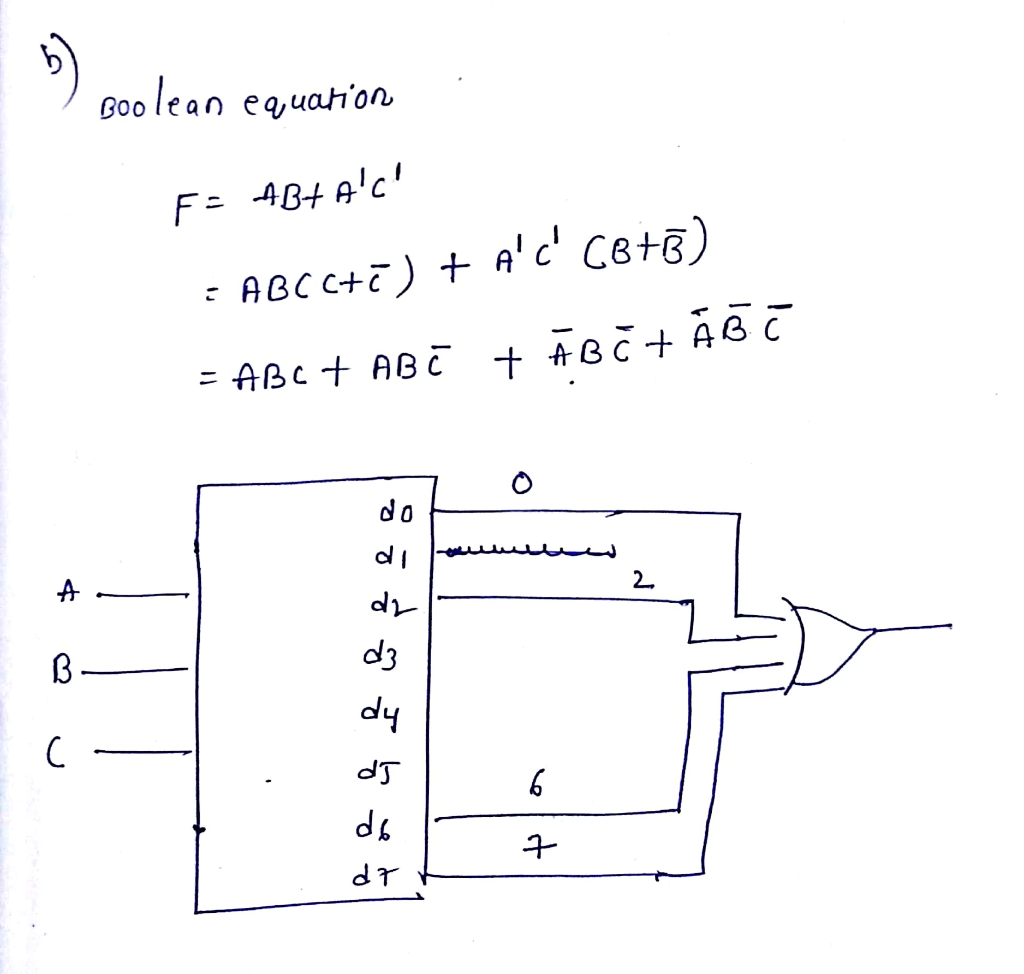Know the answer?
Your Answer:

#### Post as a guest

Your Name:

What's your source?

#### Earn Coin

Coins can be redeemed for fabulous gifts.

Not the answer you're looking for? Ask your own homework help question. Our experts will answer your question WITHIN MINUTES for Free.
Similar Homework Help Questions
• ### A combination circuit is specified by the following Boolean functions listed below. h(a, b, c) = b,c' + a'c Implement the circuit with a 3x8 decoder. Provide truth table and drawing the l...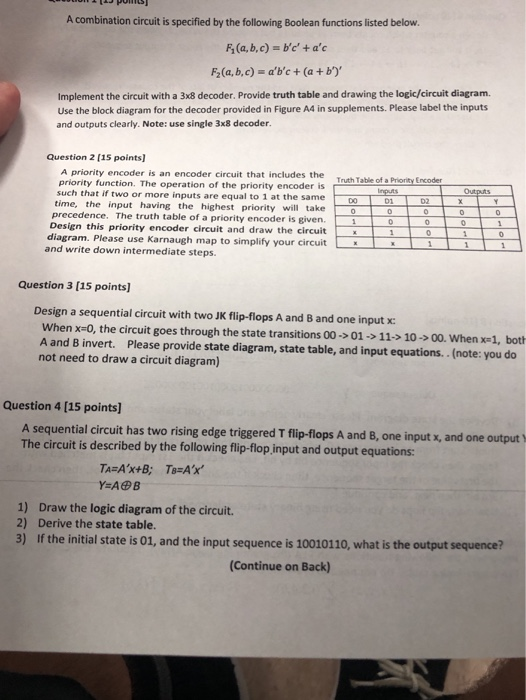A combination circuit is specified by the following Boolean functions listed below. h(a, b, c) = b,c' + a'c Implement the circuit with a 3x8 decoder. Provide truth table and drawing the logic/circuit diagram. Use the block diagram for the decoder provided in Figure A4 in supplements. Please label the inputs and outputs clearly. Note: use single 3x8 decoder Question 2 (15 points] A priority encoder is an encoder circuit that includes the Truth Table of a priority function. The...

• ### Problem 1: consider the following circuit with 4 inputs A, B, c, D, and 3 outputs...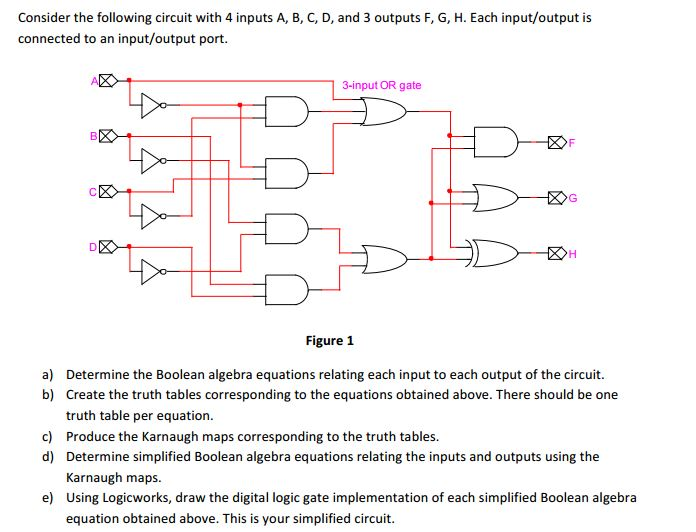Problem 1: consider the following circuit with 4 inputs A, B, c, D, and 3 outputs F, G, H. Each input/output is connected to an input/output port. 3-input OR gate Figure 1 a) Determine the Boolean algebra equations relating each input to each output of the circuit. b) Create the truth tables corresponding to the equations obtained above. There should be one truth table per equation c) Produce the Karnaugh maps corresponding to the truth tables d) Determine simplified Boolean...

• ### Acer Question Three Design a circuit with t wo inputs x & y representing the bits in a binary num...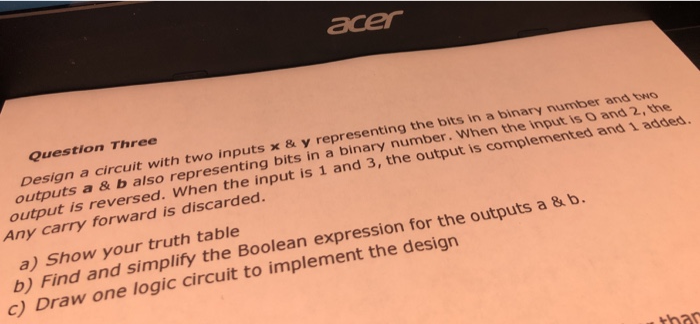acer Question Three Design a circuit with t wo inputs x & y representing the bits in a binary number and outputs a& b also representing bits in a binary number. When t output is reversed. When the input is 1 and 3, the output s O and 2, the Any carry forward is discarded a) Show your truth table b) Find and simplify the Boolean expression for the o utputs a & b. c) Draw one logic circuit to...

• ### 1.12.5 marks Design a combinational circuit (using two 8-to-1 multiplexers) with three inputs, an...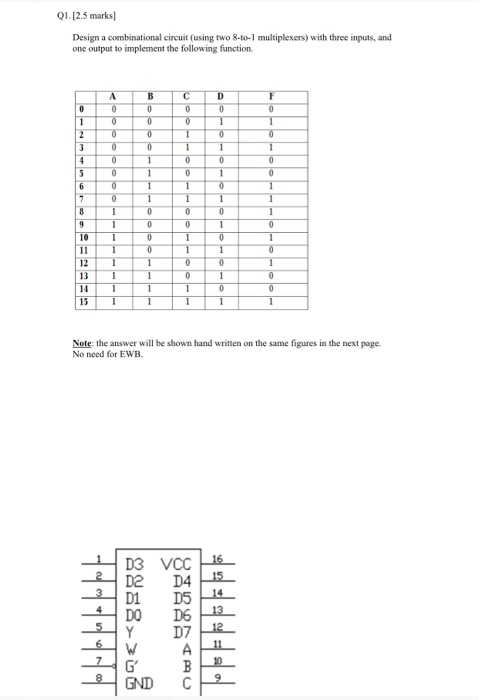1.12.5 marks Design a combinational circuit (using two 8-to-1 multiplexers) with three inputs, and one output to implement the following function 12 13 14 15 Note: the answer will be shown hand written on the same figures in the next page. No need for EWB. A I 15 Note: the answer will be shown hand written on the same figures in the next page. No need for EWB. GND C 74151 74151 1.12.5 marks Design a combinational circuit (using two...

• ### Please solve the problems from 2_5 Digital system Problem 2 Design a combinational circuit with inputs...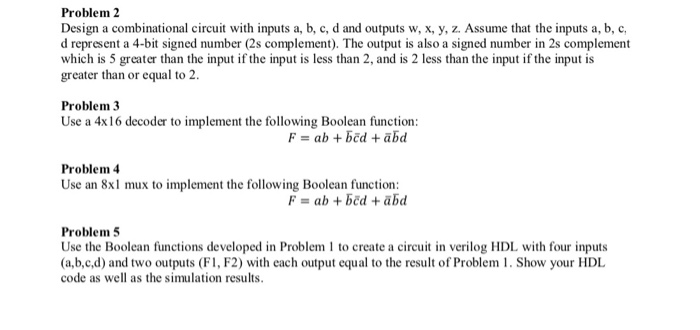Please solve the problems from 2_5 Digital system Problem 2 Design a combinational circuit with inputs a, b, c, d and outputs w, x, y, z. Assume that the inputs a, b, c d represent a 4-bit signed number (2s complement). The output is also a signed number in 2s complement which is 5 greater than the input if the input is less than 2, and is 2 less than the input if the input is greater than or equal...

• ### [2.5 marks] Design a combinational circuit (using two 8-to-1 multiplexers) with three inputs, and...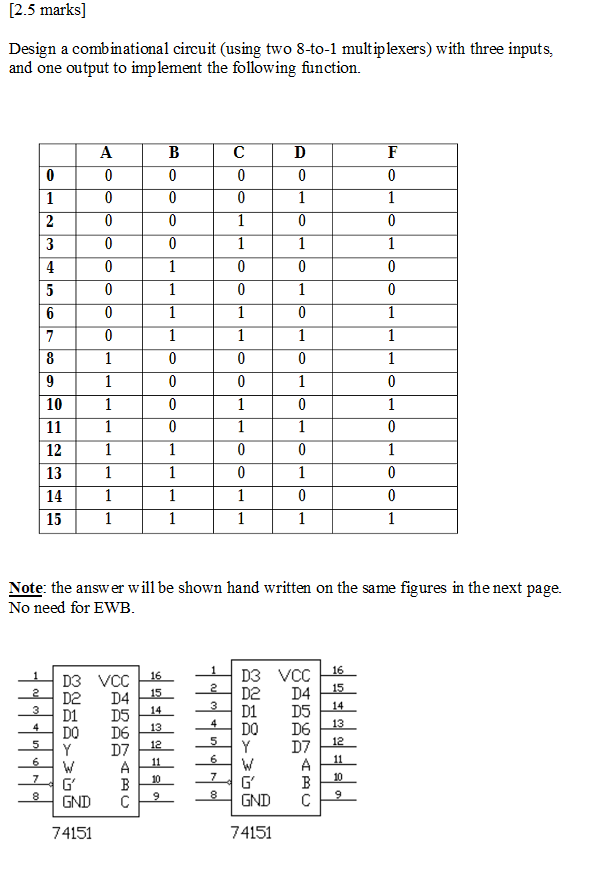[2.5 marks] Design a combinational circuit (using two 8-to-1 multiplexers) with three inputs, and one output to implement the following function. 10 12 13 14 Note: the answ er will be shown hand written on the same figures in the next page No need for EWE D3 VCC D2 D4 D1 DS DO D6 D3 VCC D2 D4 D1 D5 DO D6 GND C GND C 74151 74151 [2.5 marks] Design a combinational circuit (using two 8-to-1 multiplexers) with three...

• ###  A combinational circuit is specified by the following three Boolean function: F1(A,B,C) = {(2,4,7) F2(A,...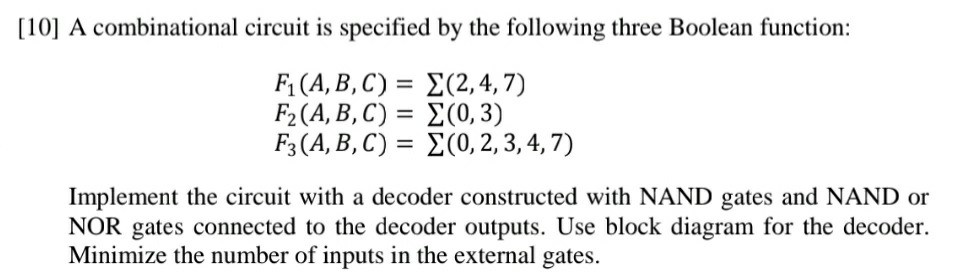A combinational circuit is specified by the following three Boolean function: F1(A,B,C) = {(2,4,7) F2(A, B, C) = 2(0,3) F3(A,B,C) = {(0,2,3,4,7) Implement the circuit with a decoder constructed with NAND gates and NAND or NOR gates connected to the decoder outputs. Use block diagram for the decoder. Minimize the number of inputs in the external gates.

• ### (0,5,6,7,11) using: Implement the circuit defined by equation F(a,b,c,d) 1. 4-to-1 multiplexers and logic gates. 2....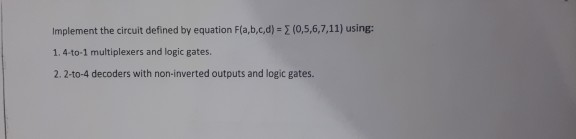(0,5,6,7,11) using: Implement the circuit defined by equation F(a,b,c,d) 1. 4-to-1 multiplexers and logic gates. 2. 2-to-4 decoders with non-inverted outputs and logic gates. (0,5,6,7,11) using: Implement the circuit defined by equation F(a,b,c,d) 1. 4-to-1 multiplexers and logic gates. 2. 2-to-4 decoders with non-inverted outputs and logic gates.

• ### 9. (Expression Truth Table) Determine the truth table for the three-input XOR function y = 11...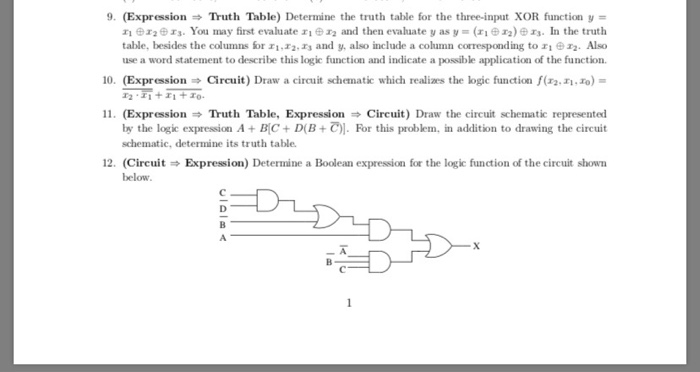9. (Expression Truth Table) Determine the truth table for the three-input XOR function y = 11 12 13. You may first evaluate i n and then evaluate y as y=( 12) 13. In the truth table, besides the columns for 11.12.13 and y, also include a column corresponding to I 1. Also use a word statement to describe this logic function and indicate a possible application of the function 10. (Expression Circuit) Draw a circuit schematic which realizes the logic...

• ### [5 pts] Design a circuit with three inputs (x,y,z) and one output that outputs true if the binary value of the inputs i...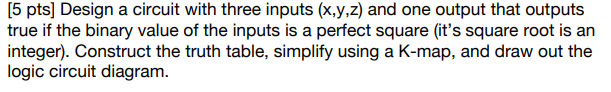[5 pts] Design a circuit with three inputs (x,y,z) and one output that outputs true if the binary value of the inputs is a perfect square (it's square root is an integer). Construct the truth table, simplify using a K-map, and draw out the logic circuit diagram [5 pts] Design a circuit with three inputs (x,y,z) and one output that outputs true if the binary value of the inputs is a perfect square (it's square root is an integer). Construct...

Free Homework App

Scan Your Homework
to Get Instant Free Answers
Need Online Homework Help?

Get Answers For Free
Most questions answered within 3 hours.